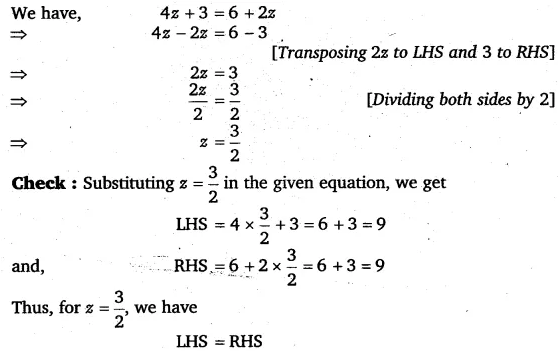# Solve the following equations and check your results: 4z + 3 = 6 + 2z

Solve the following equations and check your results:
4z + 3 = 6 + 2z